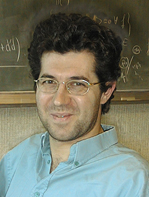# THEMATIC PROGRAMSJune  7, 2023January-June 2012 Thematic Program on Galois Representations Distinguished Lecture Series April 18-20, 2012 at 3:30 Room 230 Fields Institute Christophe Breuil Université Paris-Sud The $p$-adic Langlands program in the ordinary case and fundamental algebraic representationsThematic Program Home Page Audio of the talk Lecture Notes Distinguished and Coxeter Lecturers
 Abstract: Let $p$ be a prime number and $G$ a connected split reductive algebraic group over ${\mathbb Q}_p$ such that both $G$ and its dual $\widehat G$ have connected center. Let $\rho:{\rm Gal}(\overline {\mathbb Q}_p/{\mathbb Q}_p)\rightarrow \widehat G(E)$ be a continuous group homomorphism where $E$ is a finite extension of ${\mathbb Q}_p$. The aim of the $p$-adic Langlands program is to associate to (the conjugacy class of) $\rho$ some hypothetical $p$-adic Banach space(s) $\Pi(\rho)$ over $E$ endowed with a unitary continuous action of $G({\mathbb Q}_p)$ such that $\Pi(\rho)$ occurs in some completed cohomology groups when $\rho$ comes from some (pro)modular representation of a global Galois group. Assume that $\rho$ takes values in a Borel subgroup $\widehat B(E)\subset \widehat G(E)$. I will explain how one can associate to such a (sufficiently generic) $\rho$ a Banach space $\Pi(\rho)^{\rm ord}$ endowed with a unitary continuous action of $G({\mathbb Q}_p)$ and which is expected to be a closed subrepresentation of $\Pi(\rho)$, namely its maximal closed subrepresentation where all irreducible constituents are subquotients of unitary continuous principal series. The representation $\Pi(\rho)^{\rm ord}$ decomposes as $\Pi(\rho)^{\rm ord}=\oplus_{w\in W(\rho)}\Pi(\rho)^{\rm ord}_w$ where $W(\rho)$ is a subset of the Weyl group $W$. One important point is that its construction is directly inspired by the study of the ordinary part'' of the tensor product of the fundamental algebraic representations of $\widehat G(E)$ (composed with $\rho$). One can extend the construction of $\Pi(\rho)^{\rm ord}$ in characteristic $p$ and associate to a (sufficiently generic) $\overline\rho:{\rm Gal}(\overline {\mathbb Q}_p/{\mathbb Q}_p)\rightarrow \widehat B(k_E) \subset \widehat G(k_E)$ a smooth representation: $$\Pi(\overline\rho)^{\rm ord}=\oplus_{w\in W(\overline\rho)}\Pi(\overline\rho)^{\rm ord}_w$$ of $G({\mathbb Q}_p)$ over $k_E$ where $k_E$ is a finite extension of ${\mathbb F}_p$. When $G={\rm GL}_n$ and $\overline\rho$ comes from some modular Galois representation, I will explain how one can use recent results of Gee and Geraghty on ordinary Serre weights to prove that all ${\rm GL}_n({\mathbb Q}_p)$-representations $\Pi(\overline\rho)^{\rm ord}_w$ really do occur in spaces of automorphic forms modulo $p$ for definite unitary groups which are outer forms of ${\rm GL}_n$. The first lecture will be largely introductory, in particular I will recall the situation for $G={\rm GL}_2$ and $\rho$ reducible as above. The second lecture will be devoted to the construction of $\Pi(\rho)^{\rm ord}$ and I will stress the parallel with the restriction to subgroups of $\widehat B(E)$ of the tensor product of the fundamental algebraic representations of $\widehat G(E)$. The last lecture will be devoted to the local-global compatibility result in characteristic $p$ mentioned above. This is joint work with Florian Herzig. Christophe Breuil is one of the leading international experts on p-adic Hodge theory and the p-adic Langlands program, two of the central topics of the thematic program. In his thesis work, he introduced new p-adic Hodge theoretic techniques for studying p-adic representations of Galois groups of p-adic fields. The novelty of these techniques was that they applied to integral representations (i.e. for representations defined over Zp and not just over Qp) and to ramified p-adic fields. In 1999, Breuil joined with Brian Conrad, Fred Diamond, and Richard Taylor in applying these techniques to complete the proof of the modularity conjecture for elliptic curves over Q. The detailed computations involved in the proof of the modularity conjecture led Breuil, together with Ariane Mezard, to formulate a fundamental conjecture (now called the Breuil–Mezard conjecture) which posits an intricate relationship between p-adic Hodge theory, the representation theory of the group GL2(Zp), and the deformation theory of 2-dimensional p-adic representations of Galois groups of p-adic Galois representations. This conjecture is in some sense a quantitative local analogue of the weight part of Serre’s celebrated conjecture on modularity of mod p-representations. Taking the ideas underlying the Breuil–Mezard conjecture even further, Breuil then conjectured that there is a p-adic local Langlands correspondence relating 2-dimensional p-adic representations of the Galois group GQp and the p-adic representation theory of the group GL 2(Qp). He laid out the fundamental properties that such a correspondence would have to satisfy, and in a series of papers gave compelling evidence that this correspondence would exist. Breuil’s ideas captured the imagination of number theorists working on p-adic Hodge theory and the arithmetic of automorphic forms, and over the course of the last decade the p-adic Langlands correspondence has emerged as one of the dominant themes in this area of number theory. The p-adic Langlands correspondence for GL 2(Qp).has been constructed in general by Pierre Colmez and Vytas Paskunas. It has been used by Mark Kisin and by Matthew Emerton to provide two different proofs of the Fontaine–Mazur conjecture for odd 2-dimensional p-adic representations of GQ, with Kisin argument simultaneously establishing the Breuil–Mezard conjecture. All of this work serves to vindicate the deep and original vision of Breuil. Breuil himself continues to pursue the construction of a p-adic Langlands correspondence, with the goal of moving beyond the case of GL2(Qp) to more general contexts. He has received several prizes and honours in recognition of the importance of his contributions to his field, including giving an invited talk at the 2010 ICM in Hyderabad.

Speakers in the Distinguished Lecture Series (DLS) have made outstanding contributions to their field of mathematics. The DLS consists of a series of three one-hour lectures.